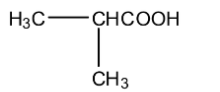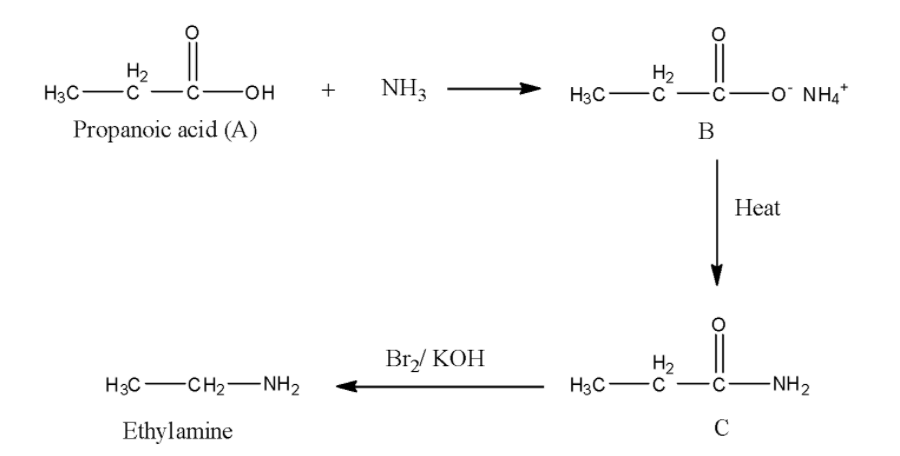QuestionAnswers

# An organic compound ‘A’ on treatment with ${\rm{N}}{{\rm{H}}_{\rm{3}}}$gives ‘B’ which on heating gives ‘C’, ‘C’ when treated with ${\rm{B}}{{\rm{r}}_{\rm{2}}}$in the presence of ${\rm{KOH}}$produces ethylamine. Compound ‘A’ is:A. ${\rm{C}}{{\rm{H}}_{\rm{3}}}{\rm{COOH}}$B. ${\rm{C}}{{\rm{H}}_{\rm{3}}}{\rm{C}}{{\rm{H}}_2}{\rm{C}}{{\rm{H}}_2}{\rm{COOH}}$ C.D. ${\rm{C}}{{\rm{H}}_2}{\rm{C}}{{\rm{H}}_2}{\rm{COOH}}$Hint: We know that Hoffmann bromamide reaction is the reaction which produces alkyl amine on reaction of amide with bromine in presence of ethanolic solution of sodium or potassium hydroxide.

In the question, it is given that reaction ‘C’ with bromine in presence of potassium hydroxide solution gives ethaylamine that means, the reaction is Hoffmann Bromoamide reaction. Then, C must be an amide. ‘C’ forms by heating of B. Then, ‘B’ must be a carboxylate ion. Then ‘A’ must be a carboxylic acid. Now, we have to determine, which carboxylic acid among the given options is correct. We know that, in Hoffmann Bromamide degradation reaction, the loss of one carbon atom takes place. In the question product formed is ethylamine, that means, the amide composed of three carbon atoms, which indicates that the carboxylic acid ‘A’ also contains three carbon atoms, that is propanoic acid.
Now, we write the whole reaction.Thus, the correct answer is option D, i.e., ${\rm{C}}{{\rm{H}}_2}{\rm{C}}{{\rm{H}}_2}{\rm{COOH}}$..

Hoffmann bromoamide reaction is a degradation reaction, where migration of an aryl or alkyl group from carbonyl carbon of amide to nitrogen atom takes place. So, the amine forms in the reaction contain one less carbon atom than that of the amide.

Note:
Students might get confused in choosing the correct carboxylic acid from the given options. They might think that, as the product of the reaction (ethylamine) consists of two carbon atoms, so the carboxylic acid also has two carbon atoms. But they must not forget that Hoffmann bromoamide reaction is a degradation reaction.

Organic Chemistry - Some Basic Principles and TechniquesSewage TreatmentAgriculture and Organic FarmingMCQ's on Chemistry in Everyday LifeReflection on A Plane MirrorDichloromethane Uses and Effects on EnvironmentCompound Interest FormulaStudy of Pollen Germination on a SlideMagnetic and Electric Force on a Point ChargeThe Difference Between an Animal that is A Regulator and One that is A ConformerImportant Questions for CBSE Class 11 Chemistry Chapter 12 - Organic Chemistry - Some Basic Principles and TechniquesNCERT Books Free Download for Class 11 Chemistry Chapter 12 - Organic Chemistry - Some Basic Principles and TechniquesImportant Questions for CBSE Class 12 Chemistry Chapter 5 - Surface ChemistryImportant Questions for CBSE Class 12 Chemistry Chapter 12 - Aldehydes, Ketones and Carboxylic AcidsNCERT Books Free Download for Class 12 Chemistry Chapter 5 - Surface ChemistryImportant Questions for CBSE Class 12 ChemistryNCERT Books Free Download for Class 12 Chemistry Chapter 12 - Aldehydes, Ketones and Carboxylic AcidsImportant Questions for CBSE Class 12 Chemistry Chapter 16 - Chemistry in Everyday lifeNCERT Books Free Download for Class 12 Chemistry Chapter 16 - Chemistry in Everyday lifeImportant Questions for CBSE Class 7 English An Alien Hand Chapter 5 - Golu Grows A NoseChemistry Question Paper for CBSE Class 12CBSE Class 12 Chemistry Question Paper 2020Chemistry Question Paper for CBSE Class 12 - 2013Chemistry Question Paper for CBSE Class 12 - 2015CBSE Class 12 Chemistry Question Paper 2019 - Free PDFCBSE Class 12 Chemistry Question Paper 2018 - Free PDFCBSE Class 12 Chemistry Question Paper 2017 - Free PDFCBSE Class 10 Hindi A Question Paper with SolutionsPrevious Year Question Paper for CBSE Class 12 Chemistry - 2014Chemistry Question Paper for CBSE Class 12 - 2016 Set 1 ENCERT Solutions for Class 11 Chemistry Chapter 12NCERT Solutions for Class 11 Chemistry Chapter 12 Organic Chemistry Some Basic Principles and Techniques In HindiSurface Chemistry NCERT Solutions - Class 12 ChemistryNCERT Solutions for Class 12 ChemistryNCERT Solutions for Class 12 ChemistryNCERT Solutions for Class 7 English An Alien Hand - A Tiger In The HouseNCERT Solutions for Class 12 Chemistry Chapter 5 Surface Chemistry in HindiNCERT Exemplar for Class 12 Chemistry Chapter-12 (Book Solutions)NCERT Solutions for Class 7 English An Alien Hand - Golu Grows A NoseChemical Kinetics NCERT Solutions - Class 12 Chemistry